Reach Us+44-1522-440391
On constructing Hermitian unitary matrices with prescribed moduli 1 | OMICS International
Journal of Generalized Lie Theory and Applications
All submissions of the EM system will be redirected to Online Manuscript Submission System. Authors are requested to submit articles directly to Online Manuscript Submission System of respective journal.

# On constructing Hermitian unitary matrices with prescribed moduli 1

Alicja SMOKTUNOWICZ1* and Wojciech TADEJ2

1Faculty of Mathematics and Information Science, Warsaw University of Technology, Plac Politechniki 1, 00-661 Warsaw, Poland,E-mail: [email protected]

2Faculty of Mathematics and Natural Sciences, Cardinal Stefan Wyszynski University, ul. Dewajtis 5, 01-815 Warsaw, Poland, E-mail: [email protected]

*Corresponding Author:
Alicja SMOKTUNOWICZ
Faculty of Mathematics and Information Science, Warsaw University of Technology,
Plac Politechniki 1, 00-661 Warsaw, Poland
E-mail: [email protected]

Received date: December 16, 2007; Revised date: March 07, 2008

Visit for more related articles at Journal of Generalized Lie Theory and Applications

#### Abstract

The problem of reconstructing the phases of a unitary matrix with prescribed moduli is of a broad interest to people working in many applications, e.g in the circuit theory, phase shift analysis, multichannel scattering, computer science (e.g in the theory of error correcting codes, design theory). We propose efficient algorithms for computing Hermitian unitary matrices for given symmetric bistochastic matrices A(n × n) for n = 3 and n = 4. We mention also some results for matrices of arbitrary size n.

#### Introduction

We will study the set of unistochastic matrices which is a subset of the set of bistochastic matrices. We say that a matrix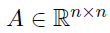is bistochastic (doubly stochastic) if all its entries are nonnegative real numbers and all its row sums and column sums are equal to 1. A unistochastic matrix is a bistochastic matrix whose entries are the squares of the absolute values of the entries of some unitary matrix U. We recall that a matrixis Hermitian if B = B*, i.e.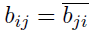for i, j = 1, . . . , n. A matrix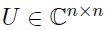is unitary if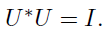A matrix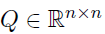is orthogonal if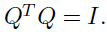We say that a symmetric bistochastic matrix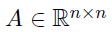is H-unistochastic if there exists a Hermitian unitary matrix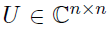such that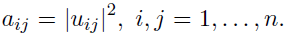If U is real (so U is orthogonal) then A is called H-orthostochastic.

Perhaps the van der Waerden matrix (Wn) is the most famous unistochastic matrix. Its elements are all equal to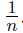. For example, for n = 4 there exists an orthogonal preimage U (2U is called an Hadamard matrix):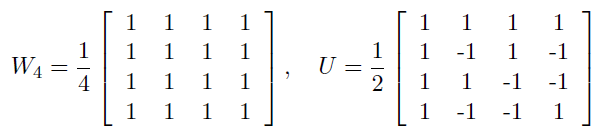so W4 is even orthostochastic (and also H- orthostochastic). However, it is easy to verify that W3 is not H-unistochastic!

Hadamard’s Conjecture (still open!) says that for n > 2 the Hadamard matrices exist when n = 4k and only for such n, see W.Tadej et al.  for explicit examples of the Hadamard matrices.

We consider the following research problems.

• I. Given a bistochastic matrix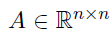check if there exists a unitary matrix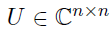such that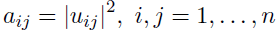(so A is unistochastic).

• II. Given a symmetric bistochastic matrix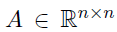check if there exists a Hermitian unitary matrix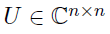such that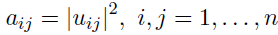(so A is H-unistochastic).

For n = 2 every bistochastic matrix A is symmetric and is orthostochastic (U can be chosen to be orthogonal). We have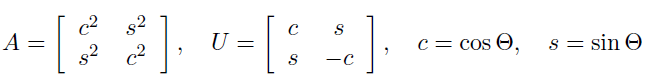Given a 3×3 bistochastic matrix A it is easy to check whether it is unistochastic or not (see e.g ). We get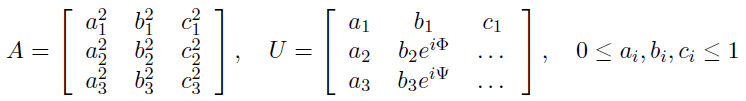Therefore the problem is to form a triangle from 3 line segments of given lengths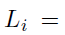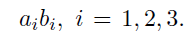Then A is unistochastic if and only if the chain-link conditions are fulfilled: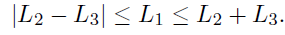In this case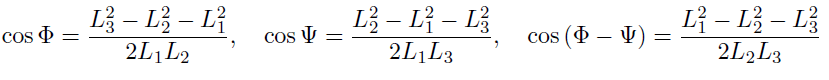Some methods for constructing unitary preimages to 3×3 bistochastic matrices are discussed in .

However, for a given 4×4 bistochastic matrix A it is not easy to check whether it is unistochastic or not! There are only partial results, so it is reasonable to try to develop efficient algorithms to check whether a given bistochastic matrix A(n × n) is unistochastic or not. We focus our attention only on symmetric bistochastic matrices and their Hermitian unitary preimages.

#### New results

For simplicity define a matrix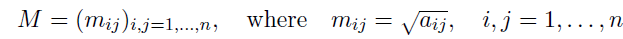Notice that without loss of generality we can seek a Hermitian unitary matrix U(n × n) in the dephased form, i.e. such that the first row and the first column of U are the same as the first row and the first column of M. It is obvious because if U is not dephased, we can find a unitary diagonal matrix D such that the matrix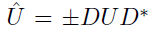satisfies these conditions and it is still Hermitian.

Given a 3×3 symmetric bistochastic matrix A it is easy to check whether it is H-unistochastic or not.

Notice that we can assume that the diagonal elements of A are ordered in such a way that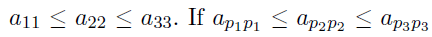for some permutation {p1, p2, p3} of {1, 2, 3}, then we can permute rows and columns of A. Define a permutation matrix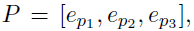where I = [e1, e2, e3]. Then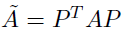has the desired property. Note also that A is H-unistochastic iff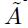is H-unistochastic. That is, U is a unitary preimage for A iff PTUP is a unitary preimage for.

As it was said above, we can assume that a Hermitian unitary U has the dephased form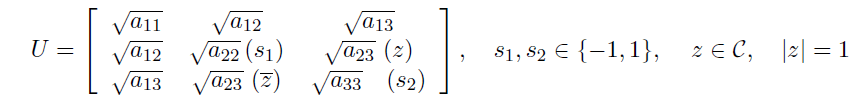Theorem 2.1. Let A(3 × 3) be a symmetric bistochastic matrix,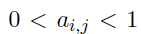for all i, j and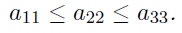Then A is H-unistochastic if and only if the following matrix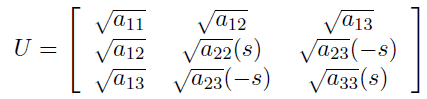where s = −1 or s = 1, is orthogonal.

Now we consider the case n = 4. Assume that all the elements of a symmetric bistochastic matrix A are nonzero. We show that our problem can be reduced to the linear system of equations.

Write A(4 × 4) and U as follows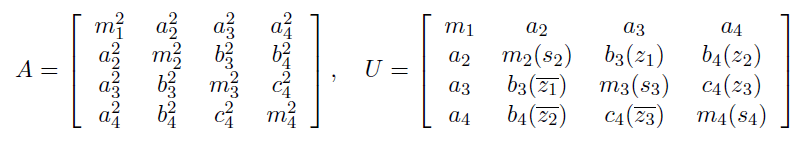where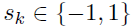and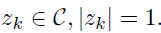Here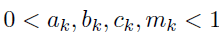is assumed in order to avoid trivial cases.

By the orthogonality of the first column of U and the columns 2, 3, 4 we obtain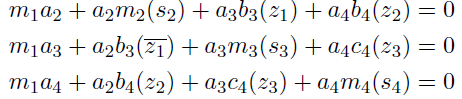We can assume that the signs sk are prescribed, in an algorithm we have to check all the combinations of signs (±1).

Let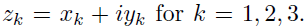Then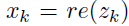can be computed as a unique solution of the following linear system of equation Bx = f, Bx = f, where x = [x1, x2, x3]T and f = [f2, f3, f4]T , where fk = −ak(m1 + mk(sk)) for k = 2, 3, 4. Here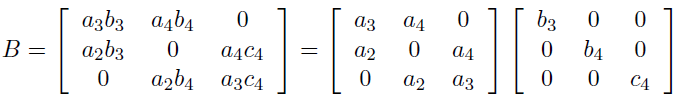Then det(B) = −2 (a2a3a4)(b3b4c4) 6= 0, so there exists a unique solution x of the linear system Bx = f. We can compute it as follows x = B−1f, where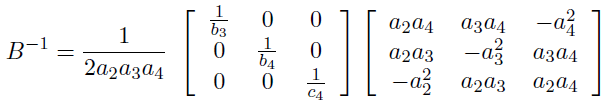Now it is easy to compute yk. We should check the conditions: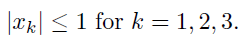Then we can compute yk from the formulae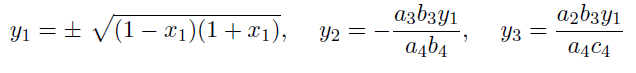Finally, we have to verify the orthogonality of the computed matrix U.

We have only some partial results for arbitrary size n. Notice that all the eigenvalues of a Hermitian unitary matrix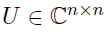are real and equal to −1 or 1. There exists a unitary matrix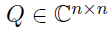such that U = QDQ*, Q*Q = I, where D = diag(1, 1, . . . , 1,−1,−1, . . . ,−1).

If we impose an additional condition on U, a Hermitian unitary preimage of A to be found, namely that U = QDQ* with Q*Q = I and D = diag(1, 1, . . . , 1,−1). Now it is not difficult to solve our problem! Notice that writing D = I − 2 diag(0, . . . , 0, 1) = I − 2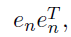we obtain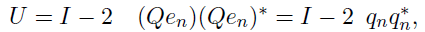so U is a reflection (Householder transformation).

Let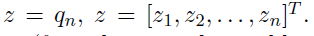Then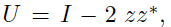where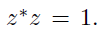We assume that a1,1 ≠ 1 (for otherwise the problem reduces to the case (n − 1) × (n − 1)). Then we can choose z1 being real and positive because U does not depend on scaling of z (if z = αu with u*u = 1 and |α| = 1, then U = I − 2 uu*). Moreover, if D is a unitary diagonal matrix then DUD* = I −2 (Du)(Du)* is also a Householder matrix, so we can search for U in the dephased form. Then the desired Householder matrix U is real and must have the following form to have the correct moduli in the first row of U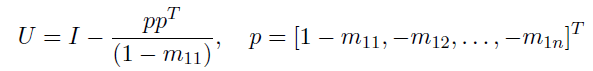Notice that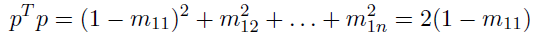so U is orthogonal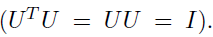The only thing to do is to compute U and check the condition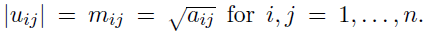the orthogonality of the columns of U we propose reorthogonalization. We apply QR decomposition to U. To compute QR decomposition we can use the Householder or Givens methods or special versions of Gram- Schmidt orthogonalization methods (see eg. ). Here is a code for MATLAB using the function qr (the Householder method):

[Q,R]=qr(U); M=A.^(1/2); Z=Q./abs(Q); U=M.*Z; I=eye(n); error_U=norm(I-U’*U)); error_A=norm(A-abs(U).*abs(U));

The numerical tests in MATLAB confirm the advantage of the proposed algorithms.

#### References

Select your language of interest to view the total content in your interested language

### Article Usage

• Total views: 11893
• [From(publication date):
September-2008 - Aug 24, 2019]
• Breakdown by view type
• HTML page views : 8090
• PDF downloads : 3803

## Post your commentCan't read the image? click here to refresh
###### Peer Reviewed Journals

Make the best use of Scientific Research and information from our 700 + peer reviewed, Open Access Journals

International Conferences 2019-20

Meet Inspiring Speakers and Experts at our 3000+ Global Annual Meetings

Top